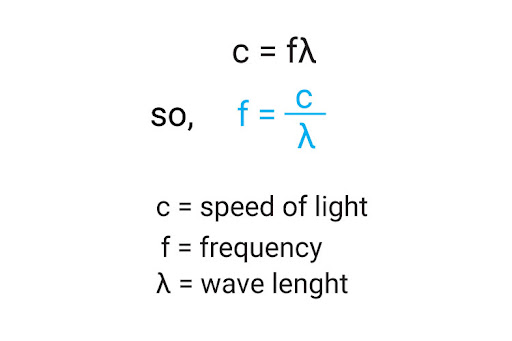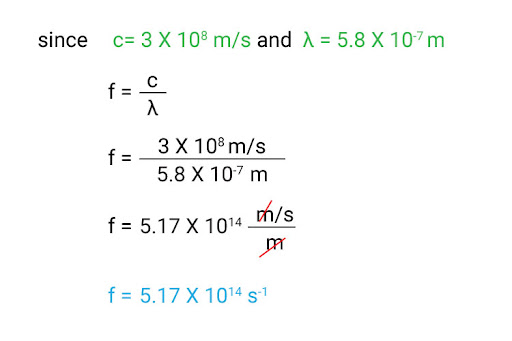# "The speed of light is 3.0 × 10^8 m/s. The wavelength of yellow light is 5.8 × 10^−7 m. Calculate the frequency of yellow light. State the unit."

A: To find the frequency, we will use the formula for wave speed:Now we will plug in the values:Twe have is s-1, or the Hertz (Hz). It helps quantify the number of complete waves made pehe unit r second.